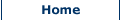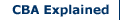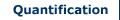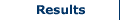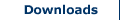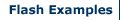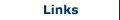Benefit Cost Ratio

The Benefit Cost Ratio can be used as a method of obtaining a result for a CBA. It provides a value by calculating the ratio of the sum of the present value of the benefits associated with a project and comparing them against the sum of the present value of the costs associated with a project. The BCR can be expressed as follows: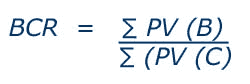Where,
BCR = benefit cost ratio
PV (B) = present value of the benefits
PV (C) = present value of the costs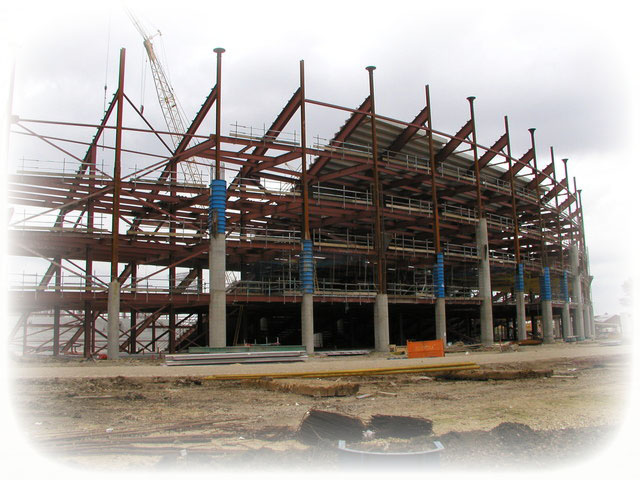It is also sometimes expressed as follows: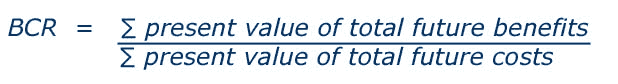In obtaining a result using BCR a researcher would interpret the BCR value as follows. It would not be rational to proceed with the project if the BCR value is below unity (1). The greater the value above unity (1), the greater the benefits associated with the alternative considered. Using BCR the researcher would choose the project with the greatest BCR.

Search CBA Builder:

•